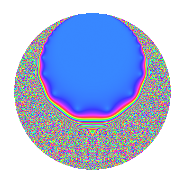# Properties

 Label 12.16.a.bLevel 12 Weight 16 Character orbit 12.a Self dual Yes Analytic conductor 17.123 Analytic rank 0 Dimension 2 CM No Inner twists 1

# Related objects

## Newspace parameters

 Level: $$N$$ = $$12 = 2^{2} \cdot 3$$ Weight: $$k$$ = $$16$$ Character orbit: $$[\chi]$$ = 12.a (trivial)

## Newform invariants

 Self dual: Yes Analytic conductor: $$17.123220612$$ Analytic rank: $$0$$ Dimension: $$2$$ Coefficient field: $$\Q(\sqrt{8017})$$ Coefficient ring: $$\Z[a_1, \ldots, a_{5}]$$ Coefficient ring index: $$2^{7}\cdot 3^{2}\cdot 5$$ Fricke sign: $$1$$ Sato-Tate group: $\mathrm{SU}(2)$

## $q$-expansion

Coefficients of the $$q$$-expansion are expressed in terms of $$\beta = 2880\sqrt{8017}$$. We also show the integral $$q$$-expansion of the trace form.

 $$f(q)$$ $$=$$ $$q$$ $$+ 2187 q^{3}$$ $$+ ( 34830 - \beta ) q^{5}$$ $$+ ( 1245752 + 9 \beta ) q^{7}$$ $$+ 4782969 q^{9}$$ $$+O(q^{10})$$ $$q$$ $$+ 2187 q^{3}$$ $$+ ( 34830 - \beta ) q^{5}$$ $$+ ( 1245752 + 9 \beta ) q^{7}$$ $$+ 4782969 q^{9}$$ $$+ ( -7487964 - 286 \beta ) q^{11}$$ $$+ ( 5878790 + 1314 \beta ) q^{13}$$ $$+ ( 76173210 - 2187 \beta ) q^{15}$$ $$+ ( 2128038642 + 1198 \beta ) q^{17}$$ $$+ ( 4620844700 - 5382 \beta ) q^{19}$$ $$+ ( 2724459624 + 19683 \beta ) q^{21}$$ $$+ ( 12127411368 + 2002 \beta ) q^{23}$$ $$+ ( 37191755575 - 69660 \beta ) q^{25}$$ $$+ 10460353203 q^{27}$$ $$+ ( 73645129206 + 79565 \beta ) q^{29}$$ $$+ ( -38338765216 + 451413 \beta ) q^{31}$$ $$+ ( -16376177268 - 625482 \beta ) q^{33}$$ $$+ ( -555076301040 - 932282 \beta ) q^{35}$$ $$+ ( -485616578194 + 307512 \beta ) q^{37}$$ $$+ ( 12856913730 + 2873718 \beta ) q^{39}$$ $$+ ( -1005351710310 + 2742314 \beta ) q^{41}$$ $$+ ( 349483151108 - 8796114 \beta ) q^{43}$$ $$+ ( 166590810270 - 4782969 \beta ) q^{45}$$ $$+ ( -391922410032 + 3658902 \beta ) q^{47}$$ $$+ ( 2190529124361 + 22423536 \beta ) q^{49}$$ $$+ ( 4654020510054 + 2620026 \beta ) q^{51}$$ $$+ ( -2792937272994 - 36041935 \beta ) q^{53}$$ $$+ ( 18757108786680 - 2473416 \beta ) q^{55}$$ $$+ ( 10105787358900 - 11770434 \beta ) q^{57}$$ $$+ ( -7918081118604 + 56238424 \beta ) q^{59}$$ $$+ ( 908137030742 - 19865196 \beta ) q^{61}$$ $$+ ( 5958393197688 + 43046721 \beta ) q^{63}$$ $$+ ( -87171254851500 + 39887830 \beta ) q^{65}$$ $$+ ( -1129033705492 - 207154152 \beta ) q^{67}$$ $$+ ( 26522648661816 + 4378374 \beta ) q^{69}$$ $$+ ( -98497659855240 - 74494550 \beta ) q^{71}$$ $$+ ( -4012928613766 + 525698208 \beta ) q^{73}$$ $$+ ( 81338369442525 - 152346420 \beta ) q^{75}$$ $$+ ( -180489377284128 - 423676748 \beta ) q^{77}$$ $$+ ( 178193033072432 + 306386217 \beta ) q^{79}$$ $$+ 22876792454961 q^{81}$$ $$+ ( 180752178743388 + 638552202 \beta ) q^{83}$$ $$+ ( -5542867449540 - 2086312302 \beta ) q^{85}$$ $$+ ( 161061897573522 + 174008655 \beta ) q^{87}$$ $$+ ( -92228154240150 + 1605980116 \beta ) q^{89}$$ $$+ ( 793707632364880 + 1689827238 \beta ) q^{91}$$ $$+ ( -83846879527392 + 987240231 \beta ) q^{93}$$ $$+ ( 518826595134600 - 4808299760 \beta ) q^{95}$$ $$+ ( -907785730540126 - 421648668 \beta ) q^{97}$$ $$+ ( -35814699685116 - 1367929134 \beta ) q^{99}$$ $$+O(q^{100})$$ $$\operatorname{Tr}(f)(q)$$ $$=$$ $$2q$$ $$\mathstrut +\mathstrut 4374q^{3}$$ $$\mathstrut +\mathstrut 69660q^{5}$$ $$\mathstrut +\mathstrut 2491504q^{7}$$ $$\mathstrut +\mathstrut 9565938q^{9}$$ $$\mathstrut +\mathstrut O(q^{10})$$ $$2q$$ $$\mathstrut +\mathstrut 4374q^{3}$$ $$\mathstrut +\mathstrut 69660q^{5}$$ $$\mathstrut +\mathstrut 2491504q^{7}$$ $$\mathstrut +\mathstrut 9565938q^{9}$$ $$\mathstrut -\mathstrut 14975928q^{11}$$ $$\mathstrut +\mathstrut 11757580q^{13}$$ $$\mathstrut +\mathstrut 152346420q^{15}$$ $$\mathstrut +\mathstrut 4256077284q^{17}$$ $$\mathstrut +\mathstrut 9241689400q^{19}$$ $$\mathstrut +\mathstrut 5448919248q^{21}$$ $$\mathstrut +\mathstrut 24254822736q^{23}$$ $$\mathstrut +\mathstrut 74383511150q^{25}$$ $$\mathstrut +\mathstrut 20920706406q^{27}$$ $$\mathstrut +\mathstrut 147290258412q^{29}$$ $$\mathstrut -\mathstrut 76677530432q^{31}$$ $$\mathstrut -\mathstrut 32752354536q^{33}$$ $$\mathstrut -\mathstrut 1110152602080q^{35}$$ $$\mathstrut -\mathstrut 971233156388q^{37}$$ $$\mathstrut +\mathstrut 25713827460q^{39}$$ $$\mathstrut -\mathstrut 2010703420620q^{41}$$ $$\mathstrut +\mathstrut 698966302216q^{43}$$ $$\mathstrut +\mathstrut 333181620540q^{45}$$ $$\mathstrut -\mathstrut 783844820064q^{47}$$ $$\mathstrut +\mathstrut 4381058248722q^{49}$$ $$\mathstrut +\mathstrut 9308041020108q^{51}$$ $$\mathstrut -\mathstrut 5585874545988q^{53}$$ $$\mathstrut +\mathstrut 37514217573360q^{55}$$ $$\mathstrut +\mathstrut 20211574717800q^{57}$$ $$\mathstrut -\mathstrut 15836162237208q^{59}$$ $$\mathstrut +\mathstrut 1816274061484q^{61}$$ $$\mathstrut +\mathstrut 11916786395376q^{63}$$ $$\mathstrut -\mathstrut 174342509703000q^{65}$$ $$\mathstrut -\mathstrut 2258067410984q^{67}$$ $$\mathstrut +\mathstrut 53045297323632q^{69}$$ $$\mathstrut -\mathstrut 196995319710480q^{71}$$ $$\mathstrut -\mathstrut 8025857227532q^{73}$$ $$\mathstrut +\mathstrut 162676738885050q^{75}$$ $$\mathstrut -\mathstrut 360978754568256q^{77}$$ $$\mathstrut +\mathstrut 356386066144864q^{79}$$ $$\mathstrut +\mathstrut 45753584909922q^{81}$$ $$\mathstrut +\mathstrut 361504357486776q^{83}$$ $$\mathstrut -\mathstrut 11085734899080q^{85}$$ $$\mathstrut +\mathstrut 322123795147044q^{87}$$ $$\mathstrut -\mathstrut 184456308480300q^{89}$$ $$\mathstrut +\mathstrut 1587415264729760q^{91}$$ $$\mathstrut -\mathstrut 167693759054784q^{93}$$ $$\mathstrut +\mathstrut 1037653190269200q^{95}$$ $$\mathstrut -\mathstrut 1815571461080252q^{97}$$ $$\mathstrut -\mathstrut 71629399370232q^{99}$$ $$\mathstrut +\mathstrut O(q^{100})$$

## Embeddings

For each embedding $$\iota_m$$ of the coefficient field, the values $$\iota_m(a_n)$$ are shown below.

For more information on an embedded modular form you can click on its label.

Label $$\iota_m(\nu)$$ $$a_{2}$$ $$a_{3}$$ $$a_{4}$$ $$a_{5}$$ $$a_{6}$$ $$a_{7}$$ $$a_{8}$$ $$a_{9}$$ $$a_{10}$$
1.1
 45.2689 −44.2689
0 2187.00 0 −223039. 0 3.56657e6 0 4.78297e6 0
1.2 0 2187.00 0 292699. 0 −1.07507e6 0 4.78297e6 0
 $$n$$: e.g. 2-40 or 990-1000 Significant digits: Format: Complex embeddings Normalized embeddings Satake parameters Satake angles

## Inner twists

This newform does not admit any (nontrivial) inner twists.

## Atkin-Lehner signs

$$p$$ Sign
$$2$$ $$-1$$
$$3$$ $$-1$$

## Hecke kernels

This newform can be constructed as the kernel of the linear operator $$T_{5}^{2}$$ $$\mathstrut -\mathstrut 69660 T_{5}$$ $$\mathstrut -\mathstrut 65283075900$$ acting on $$S_{16}^{\mathrm{new}}(\Gamma_0(12))$$.